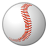PSoC™ 4Level 1Level 1

# MagSense raw count formula

Hi how can I calculate the raw count in The magsense component ? like in Capsense it is explicitly stated the formula for the raw count but I could not find any of magsense is it the same with Capsense? here is the formula of raw count in Capsense

thanks

1 SolutionEmployeeEmployee

# Re: MagSense raw count formula

Hi,

Raw count is the representative of the amplitude of sinusoidal signal that appears across tank circuit.  Based on the position of metal target, the inductance of the sensor varies which results in change in the amplitude of the sinusoidal signal across LC tank.  Hence change in the sensor inductance (due to metal position) reflects in change in raw count.  Please refer the section 3 in Inductive Sensing Design Guide (https://www.cypress.com/documentation/application-notes/an219207-inductive-sensing-design-guide ).

Now coming to your particular application of Linear Position Sensing using inductive sensor, if we combine the collective models of "Moving target position->L, L->Vamp, Vamp -> Rawcount", we will get quite non linear response.  Having said that I would recommend to use two or more point position calibration of the moving target.  The entire range of position detection can be split into pieces and have piece-wise linearization in FW to decode the position value.  Hope this helps.  Please let me know if you need any clarification.  Thank you!

Best Regards,

Dinesh

3 RepliesEmployeeEmployee

# Re: MagSense raw count formula

Hi,

Could you please let us know why you require this information? We would like to know if there is any specific use case where the raw count equation is necessary.

The change in Vamp is proportional to the change in inductance seen at the LC tank circuit.

Thanks & Regards,

ShanmathiLevel 1Level 1

# Re: MagSense raw count formula

HI thanks for answering me . I am trying to use Magsens to develop a linear position sensor. Since the Magsense does not support linear sition application, I have to decode the value of the raw count and apply it for linear position sensor. so my question is :

1) what is raw count? like is it the voltage amplitude of the LC tank or it is the inductance or current( because of the IDAC)? I am realy confused. As you answered me "The change in Vamp is proportional to the change in inductance seen at the LC tank circuit." then what is  relation between  Vamp and raw count?

2) I would like also to know the formula for raw countEmployeeEmployee

# Re: MagSense raw count formula